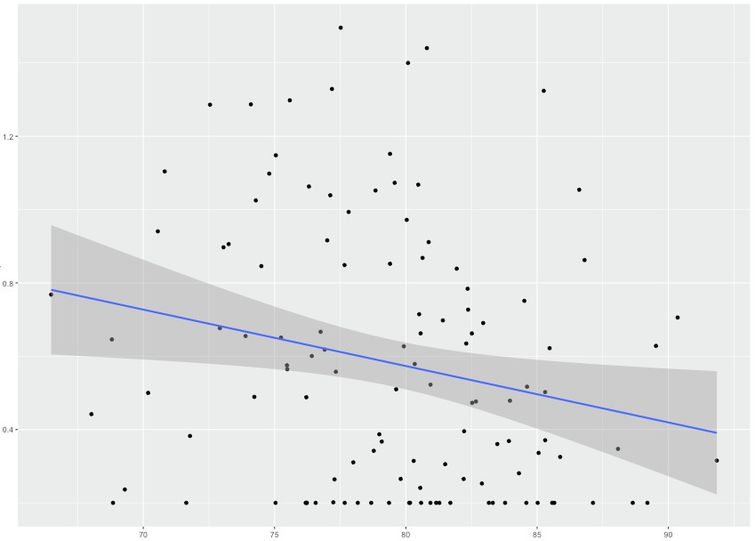Learn how to use Python to visualize metrics in Wavefront.

The Wavefront system can handle your real-time, high-frequency data by offering fast ingestion, fast querying, fast analytics, visualization, and alerting. Wavefront Query Language is capable of performing most of the transformations you’ll need for daily monitoring. However, there can be cases when you want to perform computations that the query language doesn’t currently offer, or leverage a set of libraries you’ve already written in Python to do analytics. In these cases, you can run Python as a separate analytics layer on top of your Wavefront account.

With Python and Wavefront, you can do just about any sort of analysis or visualization you can imagine. For example:

• Examine a histogram of your metric at an arbitrary bin width, or a heat map of the correlations between your metrics.
• Model your metrics for trends, seasonality, noise.
• Make a forecast about future behavior.

## Prerequisites

To use Python and Wavefront together, you need:

## Installation and Setup

To set up the Python integration,

1. Follow the instructions on the github page.
2. Install the following packages: `pip install ggplot`
3. Pull in the Wavefront Python library: `wget https://github.com/wavefrontHQ/integrations/blob/master/wavefront-python/wavefrontpython.py`
4. Import `wavefrontpython.py` in to your Python file. `from wavefrontpython import*`

## Getting Data Into Python

The Wavefront Python library allows you to perform the same queries through Python that you normally perform in a ts() query. You also have the same control over the time range. For example, you might enter `ts(requests.latency)` to grab the metric `requests.latency` over 20 hosts: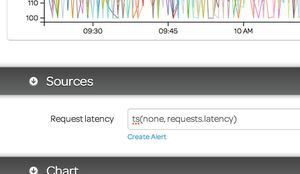In Wavefront Python you enter that query expression verbatim to retrieve the same data in numerical format, as a Python Pandas dataframe:

``````df = wfquery(base, token, wfnow() - wfhours(2), wfnow() - wfminutes(1), 'ts(requests.latency)', granularity='m')
``````

Let’s look at this query in more detail:

• The `base` and `token` variables were set in the prerequisites, and allow Wavefront to know where to query and how to authenticate that query.
• The third and fourth fields are the start and end times for the query, in epoch second format.
• The fifth field is the actual ts() query.
• The final field is granularity. It specifies the number of collections within the start and end time. Schematically, the function is:

``````df = wfquery(serverURL, wavefrontAccountToken, startTime, endTime, query, granularity)
``````

The `wfnow()` function is a convenience function for the most recent time, so the range that we’re requesting is from `wfnow() - wfhours(2)`(two hours ago) to `wfnow() - wfminutes(1)` (one minute ago). This should give us exactly 120 observations (one per minute) over all the hosts that were emitting the `requests.latency` metric.

Let’s look at the result now. The data frame returned has

• one column per returned time series
• an initial column named time, which contains the epoch seconds for that row.

For example, in the call above, the data frame has 120 (time) observations of 21 (time+host) variables; here’s the top of that data frame: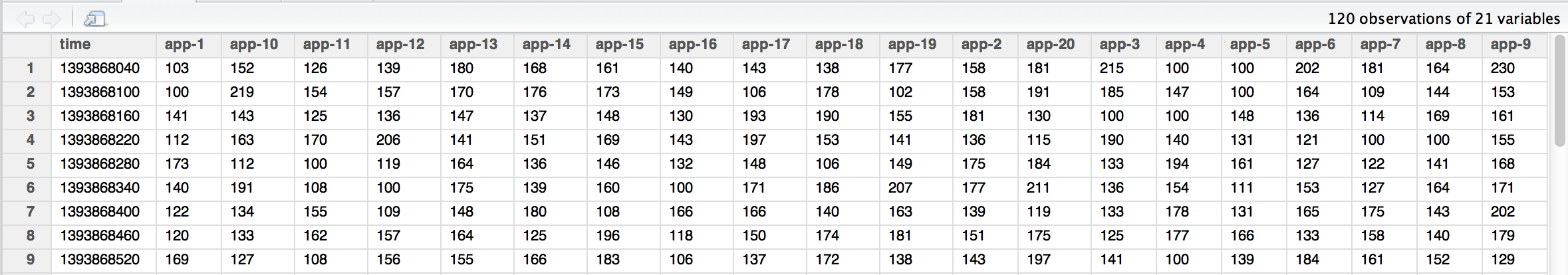In Python, you can manipulate this data frame like any other data frame. If you wanted to see the request latencies divided by 100, or an average across all of the hosts, or the data from a month ago, you would do so in exactly the same way as you do from the Wavefront web site:

``````d = wfquery(base, token, wfnow() - wfhours(2), wfnow() - wfminutes(1), 'ts(requests.latency) / 100', granularity='m')
d = wfquery(base, token, wfnow() - wfhours(2), wfnow() - wfminutes(1), 'avg(ts(requests.latency))', granularity='m')
d = wfquery(base, token, wfnow() - wfhours(2), wfnow() - wfminutes(1), 'lag("one month ago", avg(ts(requests.latency)))', granularity='m')
``````

Note that we use single quotes (‘’) around the query field, but use double quotes (“”) for internal strings such as the first argument to `lag()`). You can even divide metrics by each other and then perform functions (such as `lag`) on the result:

``````df = wfquery(base, token, wfnow() - wfhours(2), wfnow() - wfminutes(1), 'lag("one month ago", ts(requests.failures.num) / ts(requests.total.num))', granularity='m')
``````

All of the queries above look at the most recent 2 hour period of data, taken at the minute granularity. However, you can look at longer periods of data with coarser granularity. For example, here is the most recent week of data, taken at the hour granularity (`granularity='h'`):

``````df = wfquery(base, token, wfnow() - wfdays(7), wfnow(), 'ts(requests.latency)', granularity='h')
``````

The resulting dataframe has 169 observations (1 for each hour over the last week) over the 21 variables (1 time column, and 20 host columns):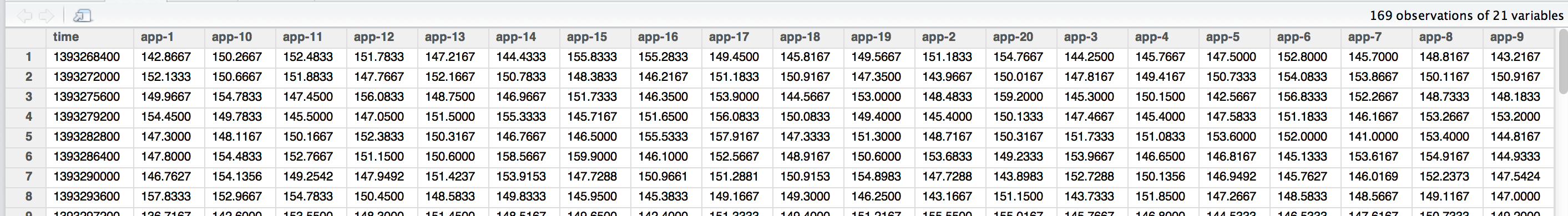The time rows are spaced apart by 3600 seconds, rather than 60 seconds. If you pick a long time range and a short granularity, the request eventually times out with no data returned. For most use cases either using a coarser granularity or using `lag()` with a short window gives you the results you need.

## Visualizing Data in Python

After you’ve gotten your Wavefront data into Python, you can do some interesting visualizations of the data.

First run a query to pull some data into a data frame:

``````# Grab requests.latency over the last 2 hours
df = wfquery(base, token, wfnow() - wfhours(2), wfnow() - wfminutes(1), 'ts(system.load5)', granularity='m')
# Remove any missing data
df = df.dropna()
``````

### Line Plot Visualization

``````# Show a line plot
d1 = df.drop('time', axis=1)
d1 = pd.melt(d1)
t1 = df['time'].tolist()
x = 1
t2 = []
while x < len(df.columns):
t2 += t1
x += 1
t2 = pd.DataFrame({'time': t2})
d2 = pd.concat([t2, d1], axis=1)
d2 = d2.dropna()
d2 = d2.rename(index=str, columns={"variable": "Linux_Host", "value": "System_Load"})
print ggplot(d2, aes('time', 'System_Load', 'Linux_Host')) + geom_line() + ggtitle("Linux Host System Load - Line plot")
``````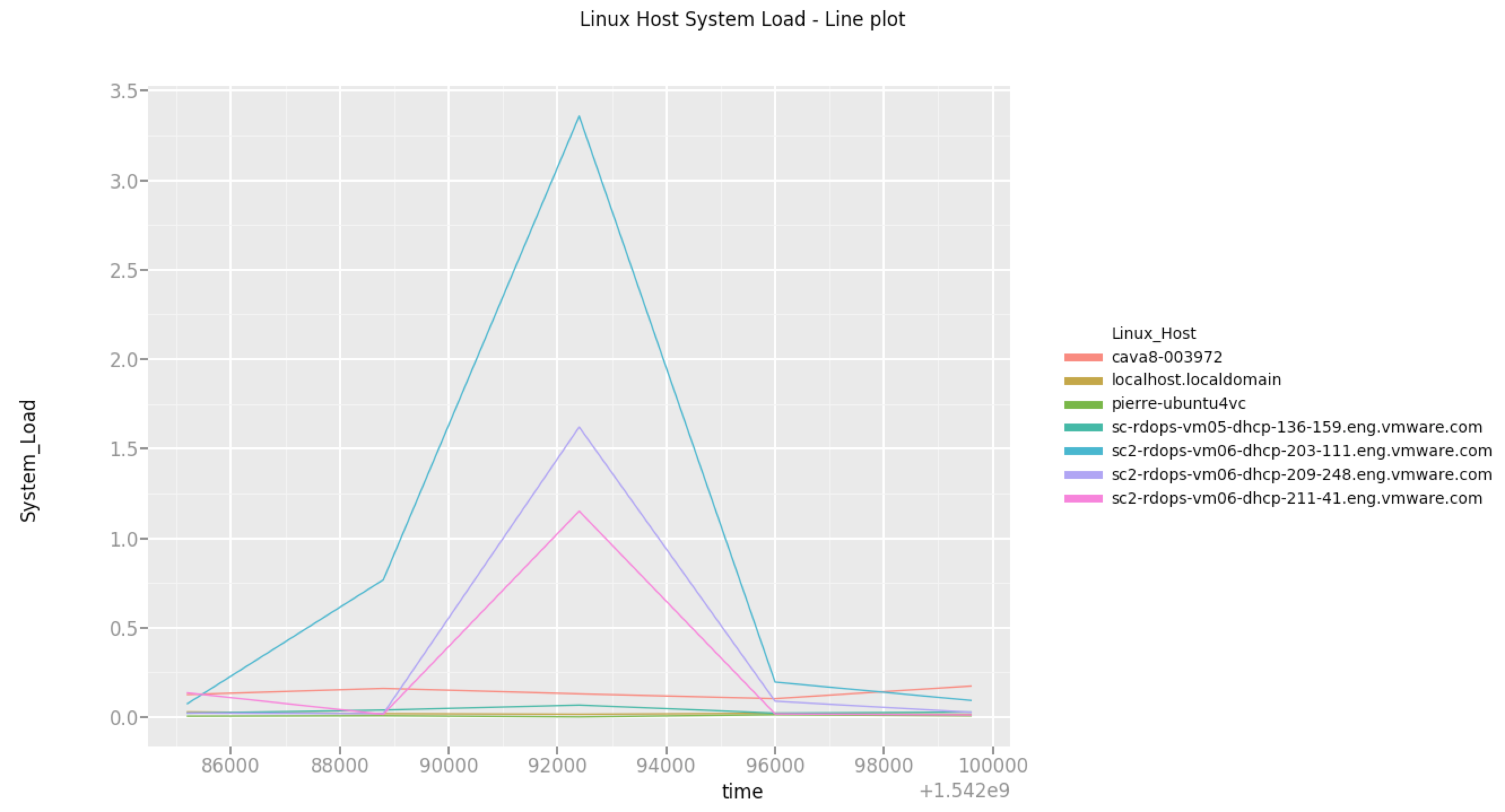### Point Plot Visualization

``````# Show a point plot
print ggplot(d2, aes('time', 'System_Load', 'Linux_Host')) + geom_point() + ggtitle("Linux Host System Load - Point plot")
``````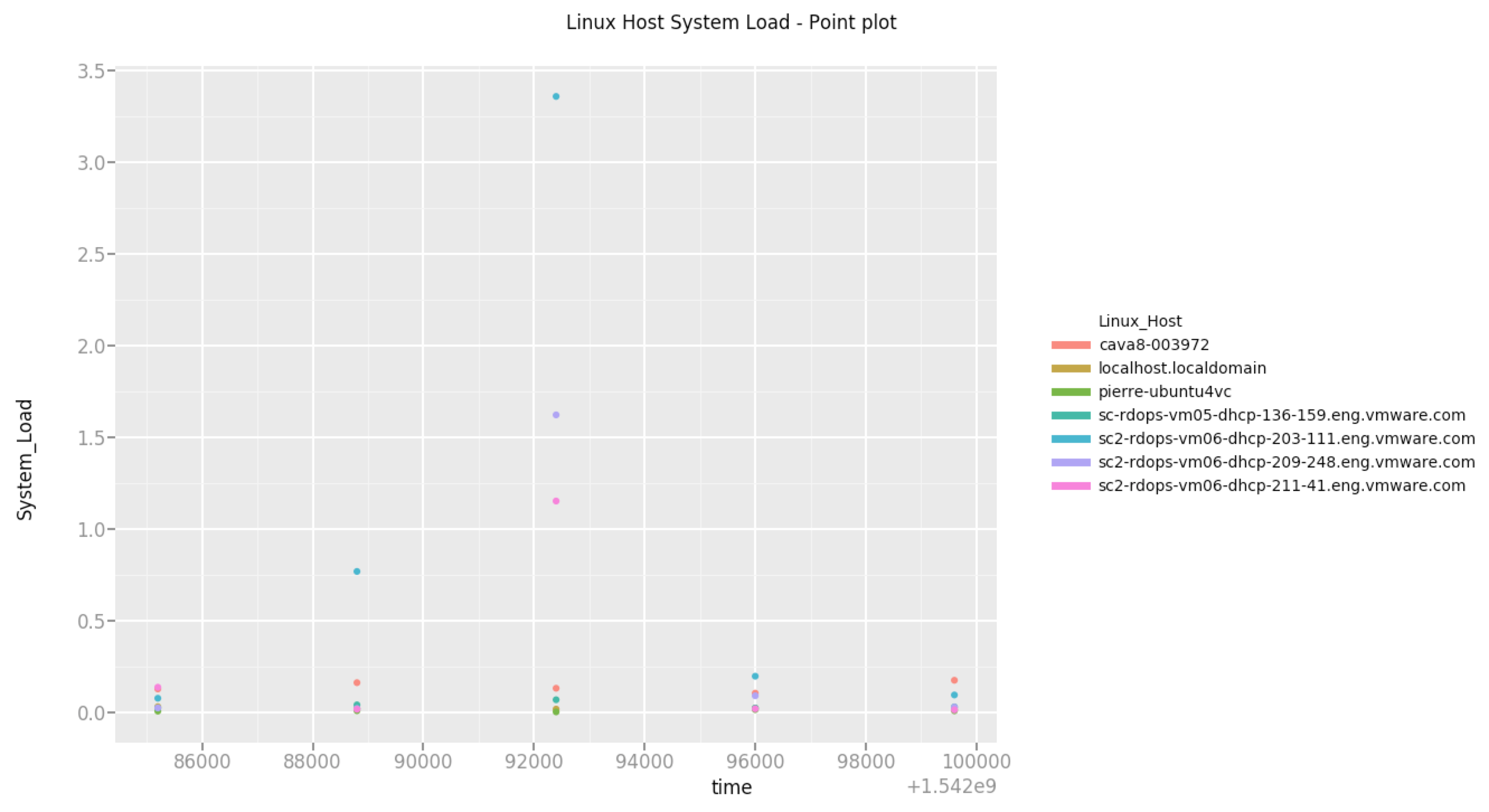### Histogram Visualization

``````# Show a Histogram
print ggplot(d2, aes(x='System_Load', fill='Host')) + geom_histogram(alpha=0.6, binwidth=2) + ggtitle("Histogram")
``````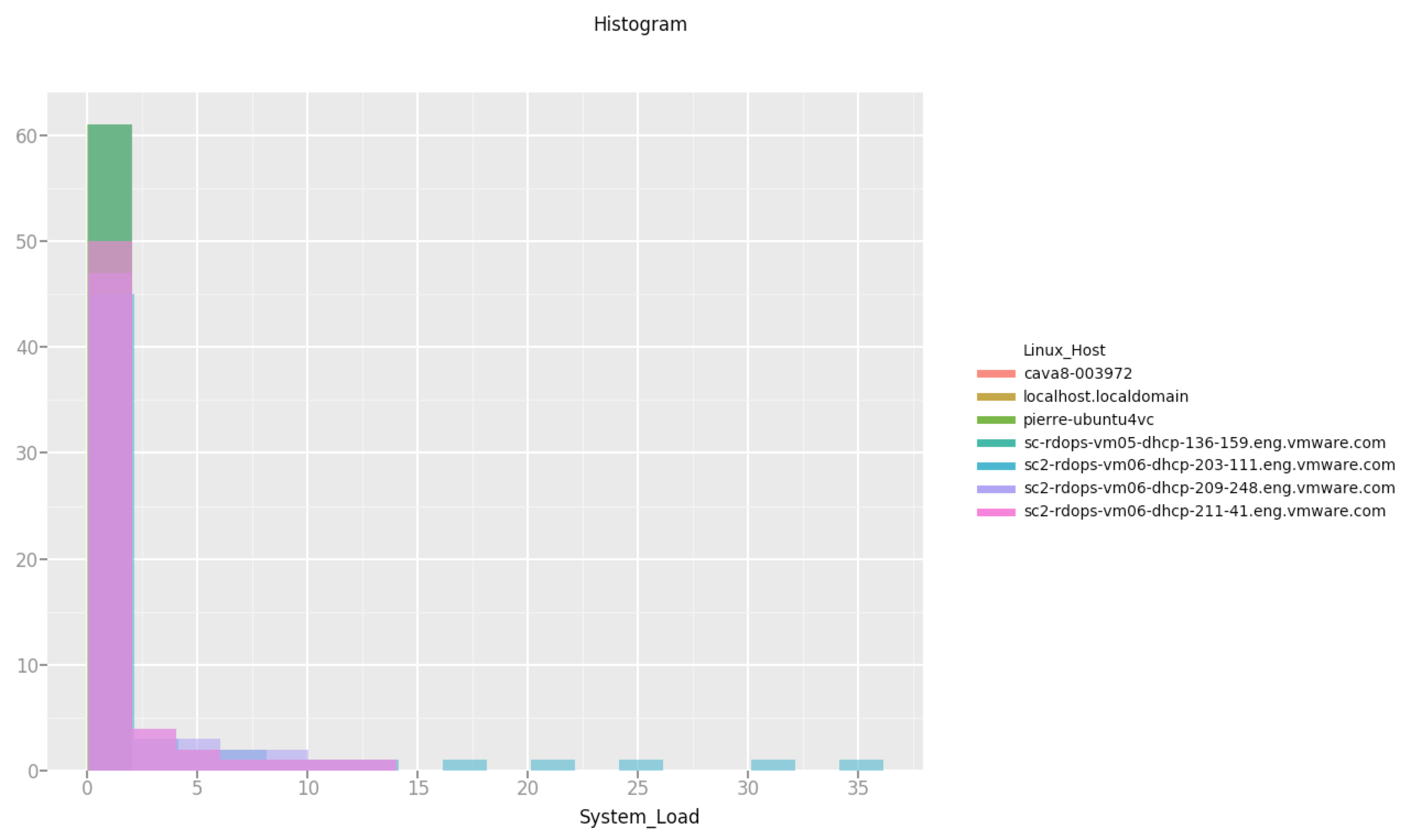## Analyzing Data in Python

Beyond visualizing data, you can use Python to perform more complicated analyses on the data than is possible within the Wavefront Query Language. Here is a linear regression example: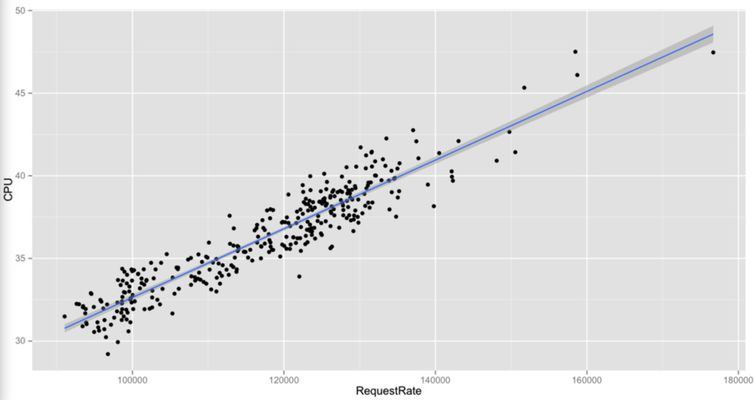``````queries = ['ts("mem.used.percentage", source=app-1)', 'ts("cpu.loadavg.1m", source=app-1)']
dataset = wfqueryvl(base,token, wfnow() - wfhours(2), wfnow() - wfminutes(1), queries, granularity='m')
scatterdata = pd.DataFrame({'time':scatterdata['time']})
scatterdata = pd.concat([scatterdata, pd.DataFrame({'Mem':scatterdata.iloc[:,1]})], axis=1)
scatterdata = pd.concat([scatterdata, pd.DataFrame({'Cpu':scatterdata.iloc[:,1]})], axis=1)
print ggplot(scatterdata, aes(x='Mem', y='Cpu')) + geom_point()
``````